Zahrada má rozměry 5m a 400cm. Kolik pletiva třeba na oplocení pozemku?

Result

x =  18 m

#### Solution:Leave us a comment of example and its solution (i.e. if it is still somewhat unclear...):Be the first to comment!#### To solve this example are needed these knowledge from mathematics:

Do you want to convert length units?

## Next similar examples:

1. Bean bag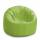A student tossed a bean bag. It landed 216 inches away. How many yards are equal to 216 inches?
2. Table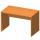Find the circumference of the table, where the long side is 1.28 meters and the short side is 86 cm.
3. RectanglesHow many different rectangles with sides integers (in mm) have a circumference exactly 1000 cm?
4. DiamondThe diamond has a circumference of 48cm. Calculate the length of its side in dm.
5. Fence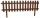How many meters of fencing it is required to purchase if our garden is rectangular measuring 20 m and 180 dm.
6. RhombusFind the length of each side of rhombus if the perimeter is 49 dm long.
7. Hr to minSue biked to school in 5/12 of an hour. How many minutes did it take her to ride to school?
8. Customary lengthConvert length 65yd 2 ft to ft
9. HoursThe lesson lasts 45 minutes. For the week, students have 18 lesson hours. How many are the actual hours?
10. Tablecloth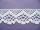The table top is 90 cm long and 50 cm wide. How many cm lace edging is necessary for the tablecloth that hangs on each side by 10 cm?
11. TailorTailor has an estimated 11 meters of 80 cm wide fabric. He will shape wholly 7 rectangles measuring 80 x 150 cm and nothing fabric does not remain. How many fabric he have?
12. Clock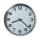How many hours are 15 days?Added together and write as decimal number: LXVII + MLXIVCalculate perimeter of the rectangle with sides a=2.4 m and b=1.9 m.Rectangular playground is fenced with 38 m long netting. Its width is 7 m. Calculate its length.A rectangular garden 40 m long and 30 m wide is to be fenced with fence posts at each corner. All the other posts will be 5 meters a part. How many posts will be needed to fence in the garden.The rectangle area is 182 dm2, its base is 14 dm. How long is the other side? Calculate its perimeter.